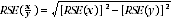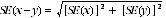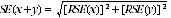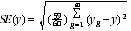4363.0.55.001 - National Health Survey: Users' Guide - Electronic Publication, 2007-08
Previous ISSUE Released at 11:30 AM (CANBERRA TIME) 14/09/2009
 Page tools: .mffeedback,#pjs { display :none; } Enable Javascript to Print PagesPrint AllEmail NotificationRSSSearch this Product This document was added 17/09/2009. STANDARD ERRORS AND REPLICATE WEIGHTS Reliability of Estimates Sample Survey Errors 1 Two types of error are possible in estimates based on a sample survey: sampling error; and non-sampling error. 2 Sampling error occurs because only a small proportion of the total population is used to produce estimates that represent the whole population. Sampling error can be reliably measured, as it is calculated based on the scientific methods used to design surveys. 3 Non-sampling error may occur in any data collection, whether it is based on a sample or a full-count (i.e. Census). Non-sampling error may occur at any stage throughout the survey process. Examples include: non-response by selected persons; questions being misunderstood; responses being incorrectly recorded; and errors in coding or processing the survey data. 4 More detailed information on sample survey errors, including sampling error, non-sampling error and response rates is provided in Chapter 7: Data Quality and Interpretation of Results. Sampling Error 5 Sampling error is the expected difference that could occur between the published estimates, derived from repeated random samples of persons, and the value that would have been produced if all persons in scope of the survey had been included. The magnitude of the sampling error associated with an estimate depends on the sample design, sample size and population variability. Measures of sampling error 6 A measure of the sampling error for a given estimate is provided by the Standard Error (SE), which is the extent to which an estimate might have varied by chance because only a sample of persons was obtained. 7 Another measure is the Relative Standard Error (RSE), which is the SE expressed as a percentage of the estimate. This measure provides an indication of the percentage errors likely to have occurred due to sampling. Standard errors of estimates of proportions 8 Proportions formed from the ratio of two estimates are also subject to sampling errors. The size of the error depends on the accuracy of both the numerator and denominator. For proportions where the denominator is an estimate of the number of persons in a group, and the numerator is the number of persons in a sub-group of the denominator population, a formula to approximate the RSE is:9 Using this formula, the RSE of the estimated proportion will be lower than the RSE estimate of the numerator. Therefore another approximation for SEs of proportions may be derived by neglecting the RSE of the denominator; i.e. obtaining the RSE of the number of persons corresponding to the numerator of the proportion and then applying this figure to the estimated proportion. Standard error of a difference 10 The difference between two survey estimates is itself an estimate, and is therefore subject to sampling variability. The sampling error of the difference between the two estimates depends on their individual SEs and the level of statistical association (correlation) between the estimates. An approximate SE of the difference between two estimates (x-y) may be calculated by the following formula:11 While this formula will only be exact for differences between separate sub-populations or uncorrelated characteristics of sub-populations, it is expected to provide a reasonable approximation for most differences likely to be of interest in relation to this survey. Standard error of a sum 12 The sum of two survey estimates is itself an estimate and is therefore subject to sampling variability. The sampling error of the sum of the two estimates depends on their individual SEs and the level of statistical association (correlation) between the estimates. An approximate SE of the sum of two estimates (x+y) may be calculated by the following formula:13 While this formula will only be exact for sums of separate sub-populations or uncorrelated characteristics of sub-populations, it is expected to provide a reasonable approximation for most estimates likely to be of interest in relation to this survey. Replicate Weights Technique 14 A class of techniques called 'replication methods' provide a general method of estimating variances for the types of complex sample designs and weighting procedures employed in ABS household surveys. 15 The basic idea behind the replication approach is to select sub-samples repeatedly from the whole sample, for each of which the statistic of interest is calculated. The variance of the full sample statistic is then estimated using the variability among the replicate statistics calculated from these sub-samples. The sub-samples are called 'replicate groups', and the statistics calculated from these replicates are called 'replicate estimates'. 16 There are various ways of creating replicate sub-samples from the full sample. The replicate weights produced for the 2007-08 NHS were created under the delete-a-group Jackknife method of replication (described below). 17 There are numerous advantages to using the replicate weighting approach, including the fact that: the same procedure is applicable to most statistics such as means, percentages, ratios, correlations, derived statistics and regression coefficients; and it is not necessary for the analyst to have available detailed survey design information if the replicate weights are included with the data file. Derivation of replicate weights 18 Under the delete-a-group Jackknife method of replicate weighting, weights were derived as follows: 60 replicate groups were formed, with each group formed to mirror the overall sample. Units from a cluster of dwellings all belong to the same replicate group, and a unit can belong to only one replicate group. For each replicate weight, one replicate group was omitted from the weighting and the remaining records were weighted in the same manner as for the full sample. The records in the group that was omitted received a weight of zero. This process was repeated for each replicate group (i.e. a total of 60 times). Ultimately each record had 60 replicate weights attached to it with one of these being the zero weight. Application of replicate weights 19 As noted above, replicate weights enable variances of estimates to be calculated relatively simply. They also enable unit record analyses such as chi-square and logistic regression to be conducted, which take into account the sample design. 20 Replicate weights for any variable of interest can be calculated from the 60 replicate groups, giving 60 replicate estimates. The distribution of this set of replicate estimates, in conjunction with the full sample estimate, is then used to approximate the variance of the full sample. 21 The formula for calculating the standard error (SE) and relative standard error (RSE) of an estimate using this method is shown below:22 where: g = (1, ..., 60) (the number of replicate weights); y(g) = estimate from using replicate weighting; and y = estimate from using full person weight. 23 The RSE(y) = SE(y)/y*100. 24 This method can also be used when modelling relationships from unit record data, regardless of the modelling technique used. In modelling, the full sample would be used to estimate the parameter being studied (such as a regression coefficient); i.e, the 60 replicate groups would be used to provide 60 replicate estimates of the survey parameter. The variance of the estimate of the parameter from the full sample is then approximated, as above, by the variability of the replicate estimates. Availability of RSEs calculated using replicate weights 25 Actual RSEs were calculated in the summary publication released for this survey. The RSEs for estimates published in the National Health Survey: Summary of Results, 2007-08 (Reissue) (cat. no. 4364.0) are available in spreadsheet format (datacubes) from the ABS web site (www.abs.gov.au). The RSEs in the spreadsheets were calculated using the replicate weights methodology. Document Selection These documents will be presented in a new window.